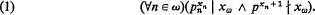Home

# A coding theorem for isols

## Extract

In  it is shown that for every sequence x = 〈xn : nω〉 ∈ Xω Λ there is an isol xω (essentially an immunized product) such thatHere we have used the notation: Λ = the isols, ω = the nonnegative integers, pn is the nth prime rational integer starting with p0 = 2, ∣ denotes divisibility and ∤ its negation. If p is an arbitrary prime, pyx, pzx, and y < z then py+1x. In particular since yω is comparable with every element of Λ, the conditions pyx and py+1x uniquely determine y. Thus every sequence xXωω is uniquely determined by an xω satisfying (1) and consequently may be used as a “code” for that sequence. In Theorem 1 it is shown that (1) does not uniquely determine the values of an arbitrary sequence xXωΛ, however in Theorem 3 we find a different scheme which does. At the very end of the paper we give some reasons why coding theorems are useful. It should also be mentioned that for a coding theorem to be meaningful it is necessary to restrict the operations by which a sequence can be recaptured from its code. Otherwise a triviality results. Our coding theorem will allow all operations which are first order definable in Λ with respect to addition, multiplication, and exponentiation. We conjecture that the latter operation is really necessary.

Hide All

# A coding theorem for isols

## Metrics

### Full text viewsFull text views reflects the number of PDF downloads, PDFs sent to Google Drive, Dropbox and Kindle and HTML full text views.

Total number of HTML views: 0
Total number of PDF views: 0 *Loading metrics...

### Abstract viewsAbstract views reflect the number of visits to the article landing page.

Total abstract views: 0 *Loading metrics...

* Views captured on Cambridge Core between <date>. This data will be updated every 24 hours.

Usage data cannot currently be displayed.